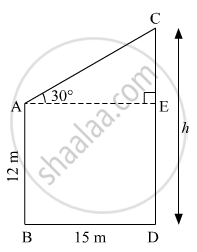Advertisement Remove all ads

# Two Buildings Are in Front of Each Other on a Road of Width 15 Meters. from the Top of the First Building, Having a Height of 12 Meter, the Angle of Elevation of the Top of the Second Building is 30° - Geometry

Advertisement Remove all ads
Advertisement Remove all ads
Advertisement Remove all ads

Two buildings are in front of each other on a road of width 15 meters. From the top of the first building, having a height of 12 meter, the angle of elevation of the top of the second building is 30°.What is the height of the second building?

Advertisement Remove all ads

#### Solution

Let AB and CD be the two building standing on the road.
Suppose the height of the second building be h m.Here, AB = 12 m, BD = 15 m, ∠CAE = 30º and AE ⊥ CD.
CE = CD − ED = (h − 12) m            (ED = AB)
AE = BD = 15 m
In right ∆AEC,
$\tan30^\circ = \frac{CE}{AE}$
$\Rightarrow \frac{1}{\sqrt{3}} = \frac{h - 12}{15}$
$\Rightarrow h - 12 = \frac{15}{\sqrt{3}} = 5\sqrt{3}$
$\Rightarrow h = \left( 12 + 5\sqrt{3} \right) m$
Thus, the height of the second building is

$\left( 12 + 5\sqrt{3} \right)$ m.
Concept: Heights and Distances
Is there an error in this question or solution?

#### APPEARS IN

Balbharati Mathematics 2 Geometry 10th Standard SSC Maharashtra State Board
Chapter 6 Trigonometry
Problem Set 6 | Q 8 | Page 139

#### Video TutorialsVIEW ALL 

Advertisement Remove all ads
Share
Notifications

View all notifications

Forgot password?